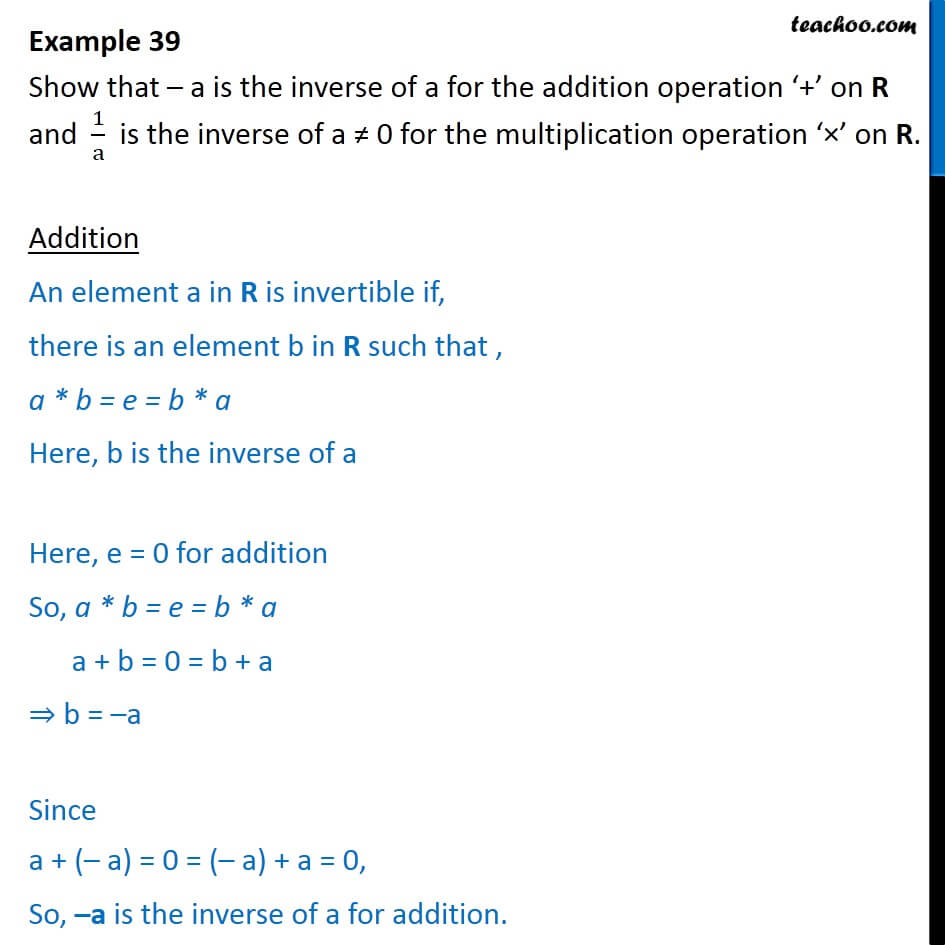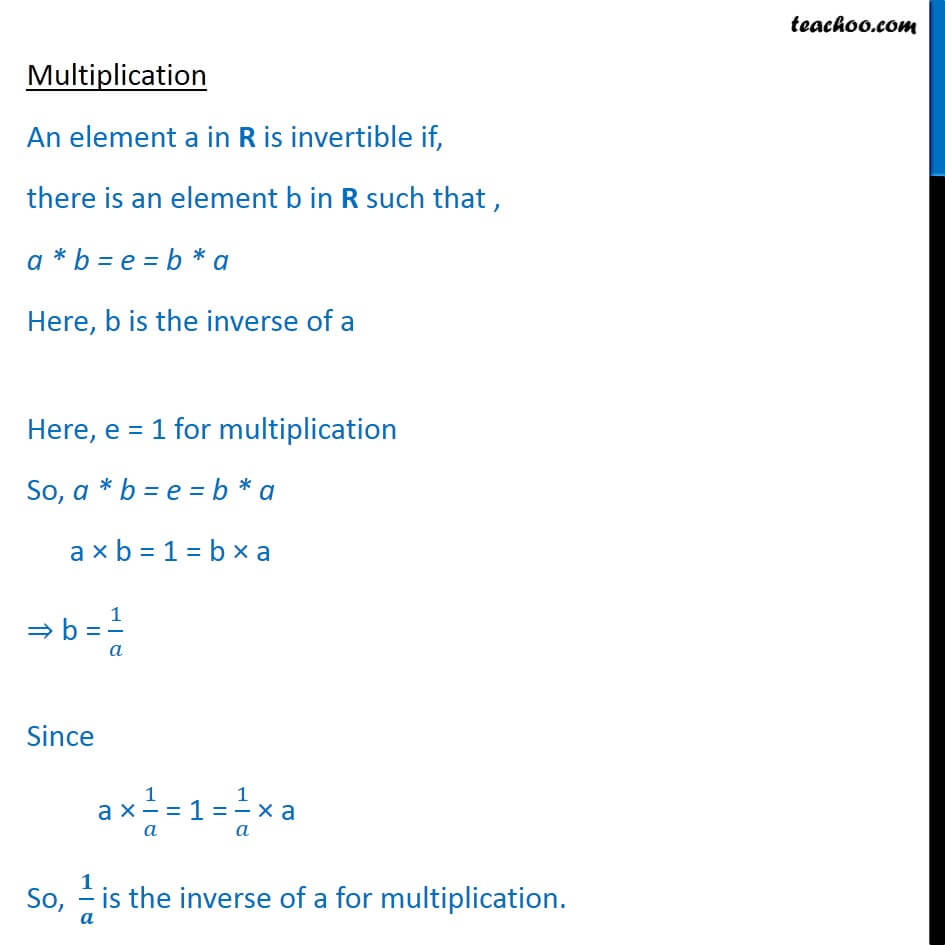1. Chapter 1 Class 12 Relation and Functions
2. Serial order wise
3. Examples

Transcript

Example 39 Show that – a is the inverse of a for the addition operation ‘+’ on R and 1﷮a﷯ is the inverse of a ≠ 0 for the multiplication operation ‘×’ on R. Addition An element a in R is invertible if, there is an element b in R such that , a * b = e = b * a Here, b is the inverse of a Here, e = 0 for addition So, a * b = e = b * a a + b = 0 = b + a ⇒ b = –a Since a + (– a) = 0 = (– a) + a = 0, So, –a is the inverse of a for addition. Multiplication An element a in R is invertible if, there is an element b in R such that , a * b = e = b * a Here, b is the inverse of a Here, e = 1 for multiplication So, a * b = e = b * a a × b = 1 = b × a ⇒ b = 1﷮𝑎﷯ Since a × 1﷮𝑎﷯ = 1 = 1﷮𝑎﷯ × a So, 𝟏﷮𝒂﷯ is the inverse of a for addition.

Examples